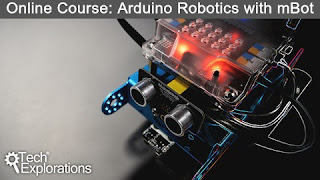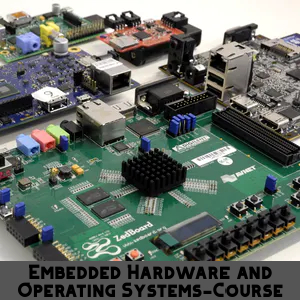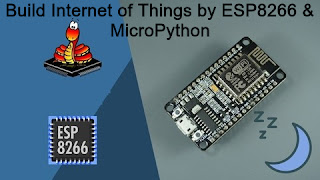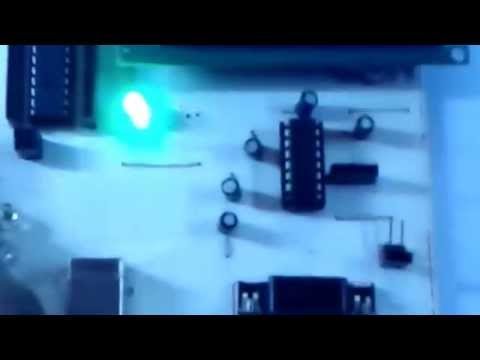# Learn Embedded Programming [Step by Step]

## Embedded Programming Tutorials for Beginners with Step By Step Guide.## Matrix [4x4] Keypad interfacing with PIC Microcontroller [ PIC18f2550 ] in Proteus [Step by Step]Matrix [4x4] Keypad  interfacing with PIC Microcontroller [ PIC18f2550 ] in Proteus [Step by Step]

Basically a matrix keypad[ 4x4] can be compared as a keyboard of microcontroller .The keypad contains 16 buttons .Internal architecture of keypad described below :Taken from Internet

You can see  the button's structure  .Here each row is connected with ground through 10k ohm resistor . Now consider that A=1, B=0,C=0,D=0 and column A get +5v .At this situation , if you press   button[A,E]  , the E link get +5v .In this way for button[A,F] , F=+5v or 1, button[A,G] , G=1, button[A,H] , H= 1.
Listen , if we can make ABCD(1000) ,ABCD(0100),ABCD(0010), ABCD(0001) through a loop . Now any button  pressed  , we can uniquely identify the button .In this way the matrix keypad works .

Now Create a project in Proteus :

### Proteus Project :

I am just trying to make easy for beginner .Now follow the instruction for pick parts from library .

Now pick  PIC18F4550  microcontroller , 22pf capacitor ,crystal , LCD DISPLAY [LM016L] , keypad  from proteus library .Now see the complete circuit given below :Matrix [4x4] Keypad  interfacing with PIC Microcontroller [ PIC18f2550 ] in Proteus [Step by Step]

Here i have connected each column with +5v instead of GND and now i am going to provide (1000) ,(0100),(0010),(0001) in each row respectively .So if any button  is pressed we get 0 according to this.
Now We have completed the Circuit and create a project in MikroC .

### MikroC Project :

If  you are a beginner , please follow the steps given below :

## Source Code :

``````

sbit LCD_RS at RB7_bit;
sbit LCD_EN at RB6_bit;
sbit LCD_D4 at RB5_bit;
sbit LCD_D5 at RB4_bit;
sbit LCD_D6 at RB3_bit;
sbit LCD_D7 at RB2_bit;
sbit LCD_RS_Direction at TRISB7_bit;
sbit LCD_EN_Direction at TRISB6_bit;
sbit LCD_D4_Direction at TRISB5_bit;
sbit LCD_D5_Direction at TRISB4_bit;
sbit LCD_D6_Direction at TRISB3_bit;
sbit LCD_D7_Direction at TRISB2_bit;
// End LCD module connections
char ch[]="   ";
int j=0,ak=0,i=0;
void main() {
CMCON=7;
TRISA.F0=1;
TRISA.F1=1;
TRISA.F2=1;
TRISA.F3=1;
TRISC.F0=0;
TRISC.F1=0;
TRISC.F2=0;
TRISC.F4=0;
Lcd_Init();
Lcd_Cmd(_LCD_CLEAR);    // Clear display
Lcd_Cmd(_LCD_CURSOR_OFF);
delay_ms(200);
j=0;
while(1){
PORTC=0x00;
ak=5;
delay_ms(200);
while (ak==5){
PORTC=0x01;       //
if(PORTA.F0==1 & PORTA.F1==0 & PORTA.F2==0 & PORTA.F3==0) {
ak=2;
Lcd_Chr(2,4,'7');
ch[j]='7';
j++;
}
if(PORTA.F0==0 & PORTA.F1==1 & PORTA.F2==0 & PORTA.F3==0) {
Lcd_Chr(2,4,'4');
ak=2;
ch[j]='4';
j++;
}
if(PORTA.F0==0 & PORTA.F1==0 & PORTA.F2==1 & PORTA.F3==0) {
Lcd_Chr(2,4,'1');
ch[j]='1';
ak=2;
j++;
}
if(PORTA.F0==0 & PORTA.F1==0 & PORTA.F2==0 & PORTA.F3==1) {
Lcd_Chr(2,4,'C');
ch[j]='C';
ak=2;
j++;
}
PORTC=0x02;
if(PORTA.F0==1 & PORTA.F1==0 & PORTA.F2==0 & PORTA.F3==0) {
Lcd_Chr(2,4,'8');
ch[j]='8';
ak=2;
j++;
}
if(PORTA.F0==0 & PORTA.F1==1 & PORTA.F2==0 & PORTA.F3==0) {
Lcd_Chr(2,4,'5');
ch[j]='5';
ak=2;
j++;
}
if(PORTA.F0==0 & PORTA.F1==0 & PORTA.F2==1 & PORTA.F3==0) {
Lcd_Chr(2,4,'2');
ch[j]='2';
ak=2;
j++;
}
if(PORTA.F0==0 & PORTA.F1==0 & PORTA.F2==0 & PORTA.F3==1) {
Lcd_Chr(2,4,'0');
ch[j]='0';
ak=2;
j++;
}
/////////////////////////
PORTC=0x04;        //
if(PORTA.F0==1 & PORTA.F1==0 & PORTA.F2==0 & PORTA.F3==0) {
Lcd_Chr(2,4,'9');
ch[j]='9';
ak=2;
j++;
}
if(PORTA.F0==0 & PORTA.F1==1 & PORTA.F2==0 & PORTA.F3==0) {
Lcd_Chr(2,4,'6');
ch[j]='6';
ak=2;
j++;
}
if(PORTA.F0==0 & PORTA.F1==0 & PORTA.F2==1 & PORTA.F3==0) {
Lcd_Chr(2,4,'3');
ch[j]='3';
ak=2;
j++;
}
if(PORTA.F0==0 & PORTA.F1==0 & PORTA.F2==0 & PORTA.F3==1) {
Lcd_Chr(2,4,'=');
ch[j]='=';
ak=2;
j++;
}
/////////////////////////
PORTC=0x10;        //
if(PORTA.F0==1 & PORTA.F1==0 & PORTA.F2==0 & PORTA.F3==0) {
Lcd_Chr(2,4,'/');
ch[j]='/';
ak=2;
j++;
}
if(PORTA.F0==0 & PORTA.F1==1 & PORTA.F2==0 & PORTA.F3==0) {
Lcd_Chr(2,4,'*');
ch[j]='*';
ak=2;
j++;
}
if(PORTA.F0==0 & PORTA.F1==0 & PORTA.F2==1 & PORTA.F3==0) {
Lcd_Chr(2,4,'-');
ch[j]='-';
ak=2;
j++;
}
if(PORTA.F0==0 & PORTA.F1==0 & PORTA.F2==0 & PORTA.F3==1) {
Lcd_Chr(2,4,'+');
ch[j]='+';
ak=2;
j++;
}
}
}
}

``````

Now follow the instructions, how to create .hex file and save .

Now go to Proteus and Run the Project .

In this way you will able to interface Keypad with Microcontroller .

## Thank You!

1.1.2.thank you, it's helped me

1.Thank you too.

3.Ain't getting any visitors!

## Featured Post

### Rs 232 , Serial Communication with PIC Microcontroller in Proteus [step by step details]## Tags

: (1) 18F2550 (1) 36KHz (3) and (1) arduino (1) Based (1) battery (1) Bipolar (1) Blinking (1) blinks (1) Bluetooth (1) button (1) circuit (1) clock (1) control (1) Db9 (1) DC Motor (2) digital (2) display (2) DS1307 (1) electronic (1) flash (1) flashing (1) HC-06 (1) home (1) how (1) How to (10) i2c tutorial (1) in (1) indicator (1) interface (8) interfacing (3) Interrupt (3) Introduction (1) IR Receiver (4) key pad (1) keyboard (1) keypad (1) lavel (1) Lcd 16x2 (2) lcd 2x16 (2) led (1) lm35 (2) LPG (1) machine (1) make (1) making (1) matrix (1) max232 (1) meter (2) microchip (4) microchips (3) mikroC (5) musical (1) NEC Protocol (4) pcb (5) PIC (3) pic controller (11) pic proteus (1) Pic Tutorial (12) pic18 (2) pic18f2550 (11) picRFモジュール (1) proteus (6) push (1) push button (1) PWM (1) real (1) Rs 232 (1) Rs232 (1) scroll (1) scrolling (1) Serial Port (1) simulation (2) step by step (7) step bystep (1) text (2) time (1) timer (4) timer0 (4) tone (1) tutorial (2) Unipolar (1) USB (1) usb 1.0 (1) USB HID (1) using (9) voltmeter (1) voting (1) with (2) work (1)

## Traffic Feed

Live Traffic Feed
Visitor Tracking